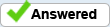# Dividing an overall number into separate components JavaScript

Hello,

I am wanting to take an overall number and divide it into a combination of numbers/check if it is even possible to do that.
So for example, someone types in 105 and the document checks if it is divisible by any combination of the numbers 15, 20, 35 and 50.
Also find the most efficient way of combining those numbers and display exactly how many of each it was divided into.
eg.
105 divided into
50 x 1
+
35 x 1
+
20 x 1

I'm wondering if this is possible and how it would be achieved, currently I have no idea where to even begin with this.
Any help is appreciated.